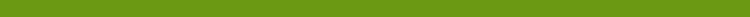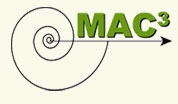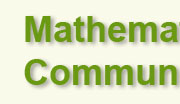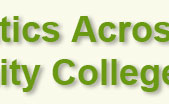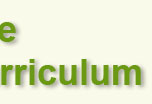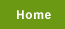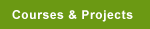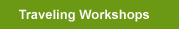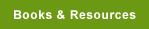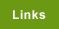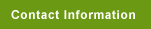MAC3 and Study Skills This project designed a coordinated studies course that consists of Math 099 (Intermediate Algebra) and Human Development (HD) 120 (Learning Strategies for Student Success). This course consists of 8 credits (5 for Math 099 and 3 for HD-120). It is anticipated that thirty to thirty-five students from two Math 099 sections will enroll in the HD-120 section. A typical section of HD-120 does not focus specifically on learning strategies for math courses. However, a special effort will be made to focus the HD-120 learning strategies in support of the Math 099 sections.   A Learning Community of College-Prep Mathematics and Student Life Skills This course integrates arithmetic and beginning algebra with the " Preparing for Student Success" course . The mathematical topics include addition, subtraction, multiplication, and division of whole numbers, fractions, decimals, and their applications; percents and applications; measurement of perimeter and area of plane figures and volumes of solids; operations on signed numbers; solving linear equations in one variable; operations on polynomials; factoring; integer exponents; radicals; graphing; simplifying algebraic fractions; applications of these topics.  In addition, this learning community will attempt to decrease students' math anxiety through exercises and activities.